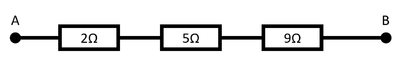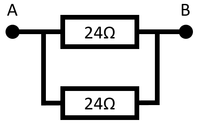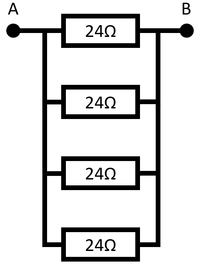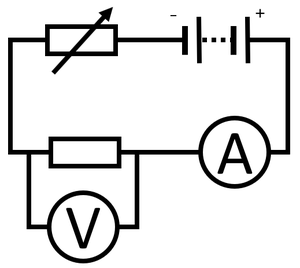# Resistor

## Key Stage 3

### Meaning

A resistor is a component in an electrical circuit which makes it difficult to electricity to pass through it.

A resistor is a component designed to have a particular resistance.
Resistors are added to circuits to control the current which will pass through for a certain potential difference.
The greater the resistance of a resistor the smaller the current will be.
The resistance of a resistor can be found by attaching an ammeter in series with the resistor and a voltmeter in parallel with the resistor.
All components in a circuit act as resistors, including wires. The longer a wire the more resistance it will have to a current.

## Key Stage 4

### Meaning

A resistor is a component in an electrical circuit which makes it difficult to electricity to pass through it.

A resistor is a component designed to have a particular resistance.
Resistors are added to circuits to control the current which will pass through for a certain potential difference.
The greater the resistance of a resistor the smaller the current will be.
The resistance of a resistor can be found by attaching an ammeter in series with the resistor and a voltmeter in parallel with the resistor.
All components in a circuit act as resistors, including wires. The longer a wire the more resistance it will have to a current.

### Resistors in Series

Adding resistors in series increases the resistance in the circuit.
The resistance of each resistor can be added together.The resistance between points A and B is: 5Ω.The resistance between points A and B is: 2Ω + 5Ω = 7ΩThe resistance between points A and B is: 2Ω + 5Ω + 9Ω = 16Ω

### Resistors in Parallel

NB: You only need to know what happens with identical resistors in parallel.

When identical resistors are added in parallel there are more paths for the electricity so the resistance is reduced.Two identical resistors in parallel gives twice the number of paths, so has half the resistance. The resistance between points A and B is 12Ω. Three identical resistors in parallel gives three times the number of paths, so has a third of the resistance. The resistance between points A and B is 8Ω. Four identical resistors in parallel gives four times the number of paths, so has a quarter of the resistance. The resistance between points A and B is 6Ω.

### IV Graph

#### Description

The IV Graph for a resistor shows that:

#### Explanation

Resistors obey Ohm's Law so the "current through a resistor at constant temperature is directly proportional to the potential difference across the resistor."

#### Obtaining the IV GraphConnect an ammeter in series with the resistor to measure current through the resistor. Connect a voltmeter in parallel with the resistor to measure the potential difference across it. Use a variable resistor in series with the resistor to vary the potential difference across the resistor. Start with a potential difference of zero and increase the potential difference by an interval of 0.2V up to 2V. Recording the reading on the voltmeter and ammeter. Reverse the connections on the battery and repeat steps 4 and 5 to find the I-V relationship for negative potential difference and current.